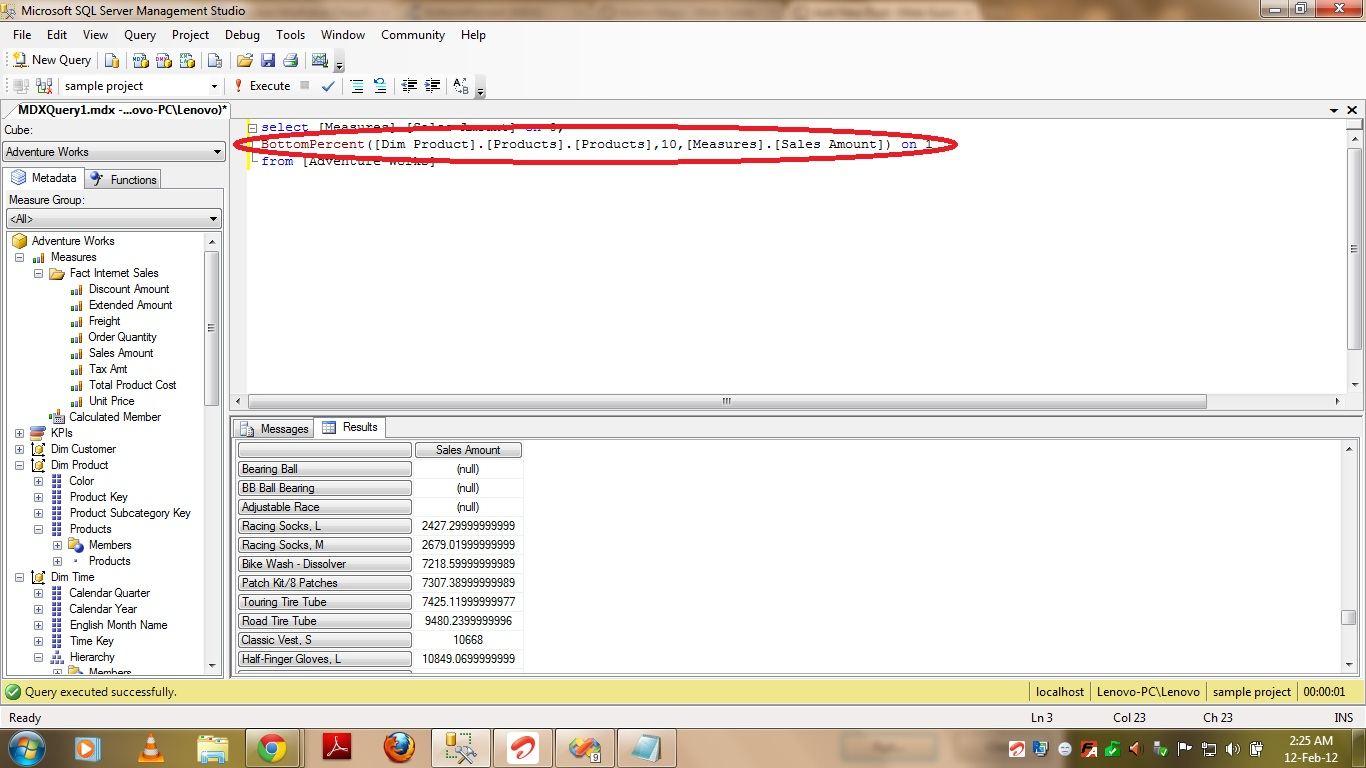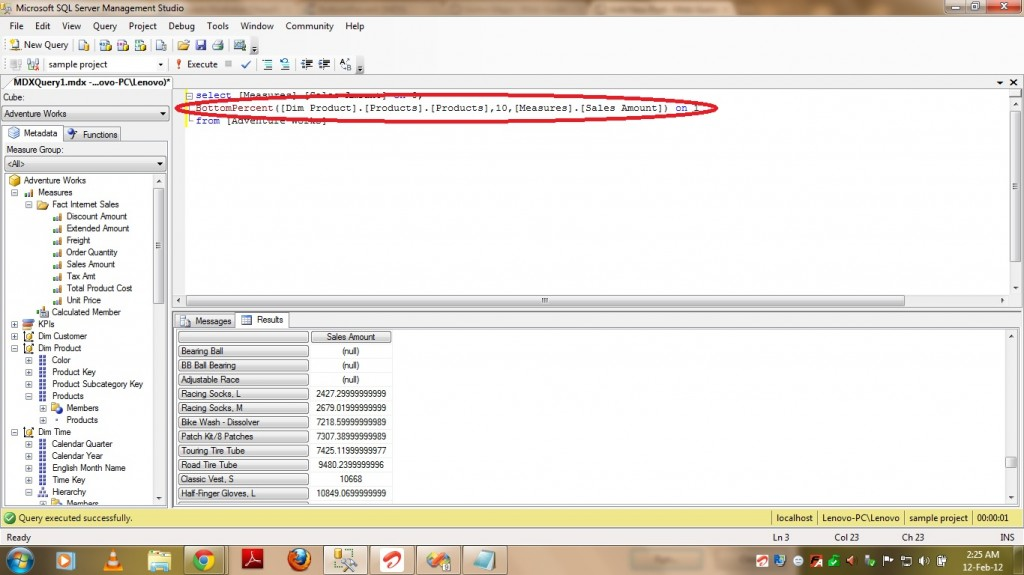# BottomPercent in MDX

4937Friends,

This funcmtion is very much similary to the functions BottomSum and BottomPercent. Please find the Major Difference between the all three functions –

1) BottomCount – Orders the set and then returns specified number of tuples in the specified set with the lowest values will be pulled.

2) BottomPercent – Orders the set and then returns tuples from the bottom of the set with the lowest values whose cumulative total is less than or equal to the supplied percentage.

3) BottomSum – Orders the set and then returns the summed value of a numeric expression across a supplied set.

In this post we are gonna discuss about BottomPercent Function. This function will do TWO jobs

1) Sorts the set based on numeric parameter in Ascending order.

2)  Returns tuples from the bottom of the set with the lowest values whose cumulative total is less than or equal to the supplied percentage.

Syntax –

BottomCount(Set_Exp,Percentage,Numeric_Exp)

Set_Exp – Valid MDX Set Expression

Count – It specifies the Percentage of Cumulative sum of records to be returned.

Numeric_Exp – It specifies on what column basis the set needs to be ordered.

The BottomPercent function sorts a set in ascending order, then returns tuples from the bottom of the set with the lowest values whose cumulative total is less than or equal to the supplied percentage. This function returns the smallest subset of a set whose cumulative total is at least the specified percentage. The returned elements are ordered largest to smallest.

PFB sample query Which pulls 10 Percent of Products based on Sales Amount.

select [Measures].[Sales Amount] on 0,
BottomPercent([Dim Product].[Products].[Products],10,[Measures].[Sales Amount]) on 1If you see the above screenshot, It is returning soo many records among which most are having NULL as Sales Amount. As we are asking for Bottom Percent of Cumulative sum of records based on Sales Amount, it is pulling all the  NULL records and PFB the MDX query to eliminate NULLs from output –

select [Measures].[Sales Amount] on 0,
BottomPercent(Filter([Dim Product].[Products].[Products],
NOT ISEMPTY([Measures].[Sales Amount])),10,[Measures].[Sales Amount]) on 1